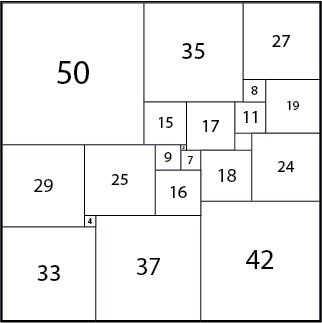I’m not keen on square designs. Something in my brain yearns after the greater dynamism of the rectangle. I like proportions of A4 paper and its larger and smaller kindred, where the relationship between the sides is the square root of 2. And of course there is the much abused ‘golden ratio’, often misapplied far beyond the relationship between shapes, as in the ungainly Fibonacci spiral.

But one square design fascinated me from the moment I first came across it – the squared square.  A squared square is the solution to a challenge which at first sight seems so simple:

Make up a square composed of squares, each of which is different in size.

You will struggle – in fact the whole of the mathematical world struggled. It appears that the first squared square was discovered, or at least announced, in 1939. Over the years that followed various of these ‘simple perfect squared squares’ were discovered. The more squares involved in the construction, the easier they were to find.

It was not until 1978 that a square constructed of 21 smaller squares was discovered and proved to be both unique and the smallest possible in terms of the number of squares used in its construction.  As a shape it just calls out for colour but every attempt ran up against the fact that it is squareAnd then the blindingly obvious struck me. If the square is stretched in one dimension it becomes a rectangle made up of ‘self-similar’ rectangles and more importantly it is subject to the same constraints as the construction of a square

Constructing a rectangle of smaller rectangles with the same aspect ratio, each different in size and in the same orientation as the large rectangle, follows the same rules as a square with the same number of elements. A rectangle constructed of self-similar rectangles, each of a different size, can be uniquely constructed of 21 elements and no less. Equally, any rectangle constructed of self-similar rectangles in the same orientation can be rescaled to be square.

So this 21-rectangle design is the simplest rectangle of this particular aspect ratio that can be constructed – and at last I can colour it without my internal disgust meter hitting the stopper.